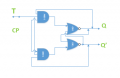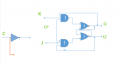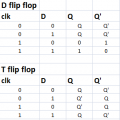# T and D flip flop together

#### Leite33

Joined Nov 28, 2015
57
Hi friends
I want again your help. I create the above flip flop circuit. Its a T flip flopSo i try to create in one flip flop circuit by using a jk flip flop and some logic gates. This flip flop will have 2 inputs. When the value of C input will be 0 flip flop will work as T flip flop. When the C input will be 1 the flip flop circuit will work as D flip flop. I am not sure how i can do that and combinate T and D flip flop by using JK flip flop

#### hp1729

Joined Nov 23, 2015
2,304
J-K flip flops are a lot more complicated than your example.

#### Attachments

• 46.7 KB Views: 2

#### WBahn

Joined Mar 31, 2012
28,484
You need to be consistent. First you talk about the behavior when the C input is this or that and now you are talking about the behavior when the S input is this or that. Which is it. Also, the statement, "When the value of S input will be 0 and as a D flip flop when the value will be 1," is nonsensical.

Start with a truth table showing the inputs you have and the behavior you want at the outputs for each input for each possible state of the flip flop.

#### Leite33

Joined Nov 28, 2015
57
Yes sorry i meant that when the value of C will be 0 then circuit will behave like T flip flop and when the C input will be 1 then the circuit will behave like D flip flop.
I think the truth table will have inputs
C TD
and outputs
Q Q' for T flip flop and for example
X X' for D flip flop.
I am not sure at all how will be the truth table

#### WBahn

Joined Mar 31, 2012
28,484
Your flip flop will only have one set of outputs -- Q and Q'. The relationship between that pair of outputs and the input will depend on the value of the C input.

First, what is the truth table for a T flip flop. Then what is the truth table for a D flip flop. Then merely stack the two truth tables on top of each other using the C input to dictate which one applies.

#### Darrell Teegarden

Joined Sep 8, 2015
25

#### Leite33

Joined Nov 28, 2015
57
I dont understand you completly . I think i have to start by creating a truth table. But i dont know how to built that table. I think the circuit of j-k flip flop i gave is correct. Now i have to use it with some logic gates to create a use of T flip flop and D flip flop together. When the C input is 0 i have T flip flop and when is 1 i have D flip flop

#### WBahn

Joined Mar 31, 2012
28,484
What is the truth table for a D flip flop? If you don't know, then LOOK IT UP!

What is the truth table for a T flip flop? If you don't know, then LOOK IT UP!

#### Leite33

Joined Nov 28, 2015
57
Hi againI thought to start design my flip flop to understand better. So my input is C and could be C=0 or C=1. When will be 0 i take the orange road and try to work it as T flip flop. When C=1 i take the blue road and work it as D flip flop. Now i put the jk flip flop above and try to make my combination. The exercise says that i have only one another input with the name TD.
My truth table of T flip flop and D flip flop i think is the above#### WBahn

Joined Mar 31, 2012
28,484
Go back and study the behavior of a T flip flop some more.

Also, these are edge triggered, so the only time they change state is upon the rising edge of the clock.

Your TD input acts like a T input when C=0 and it acts like the D input when C=1.

#### Leite33

Joined Nov 28, 2015
57
I found them so difficult. My biggest problem is that i dont have time to study more.
So in T flip flop when the clock is 0 the circuit dont work and nothing change. But when D is 1 and not 0 i take Q' and Q i got that . So the truth table isnt right? for t flip flop?

#### WBahn

Joined Mar 31, 2012
28,484
How about looking at the description of a T flip flop?

A link to Wikipedia is often a good jumping off point.

#### Leite33

Joined Nov 28, 2015
57
Hi i learnt something about flip flops. I need little help in that point that i have 2 inputs one is general input of flip flop and the other is the input that if its 0 circuit work as T flip flop and if its 1 circuit work as D flip flop. But i need to use only one JK flip flop and logic gates

#### WBahn

Joined Mar 31, 2012
28,484
Still waiting for a correct truth table from you.

This is a simple problem.

You have two inputs, Mode and Data (what you have sometimes calls C and TD). You have one state variable, Q.

So you have three variables you are concerned about, Mode, Q, and Data. That results in eight possible combinations. All you have to do is design what value Q needs to take on after the next rising clock edge for each possible combination.

What is SO hard about coming up with a truth table that has eight entries in it?

Once you have the truth table, then for each row you just need to come up with the values needed at the J and K inputs to make that row do what you want.

Instead, you are continuing to just spin your wheels in search of "a happening" -- which means you are making almost random attempts hoping that, at some point, something good will just "happen" to work.

•absf